Function Repository Resource:

# PhysicalQuantityData

Calculate data related to physical quantities

Contributed by: Peter Cullen Burbery
 ResourceFunction["PhysicalQuantityData"][] lists every physical quantity. ResourceFunction["PhysicalQuantityData"][physicalquantity, property] calculates the value of the property for physicalquantity.

## Details

ResourceFunction["PhysicalQuantityData"] is a convenient short cut for "PhysicalQuantity” Entity values.
Supported properties include: "Abbreviations", "AlgebraicTypes", "AlternateNames", "BasePhysicalQuantity", "CanonicalUnit", "Classes", "Dimensions", "EntityClasses", "Instances", "Name", "NamedSIUnit", "PhysicalPropertyType", "QuantityVariable", "SIBaseUnit", "SIUnit", "StandardIdentifiers", "StandardSymbols" and "Symbols".
“PhysicalPropertyType" determines whether a quantity is extensive. Extensive properties are dependent on the amount of a substance but intensive properties are the same no matter how much you have of something. For example, density is constant but the mass and volume of an object changes:

## Examples

### Basic Examples

Find the SI base unit for magnetic inductance:

 In:=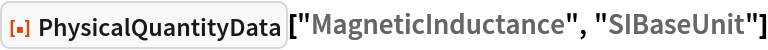Out=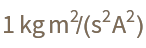Compute extensive and intensive properties of the SI base quantities:

 In:=In:=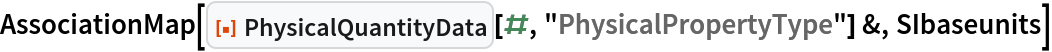Out=Select intensive values:

 In:=Out=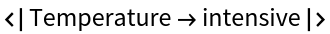Get the base units:

 In:=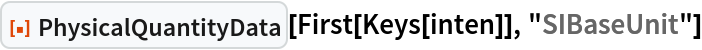Out=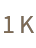Find instances of time:

 In:=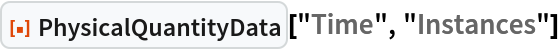Out=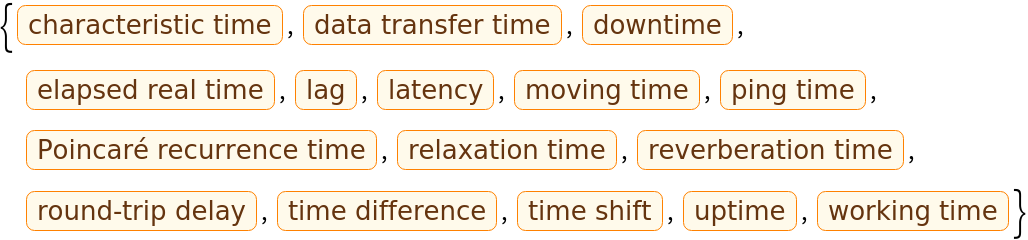Display the instances as a column of strings:

 In:=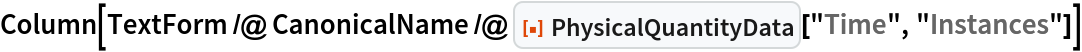Out=Display instances of mass in a column:

 In:=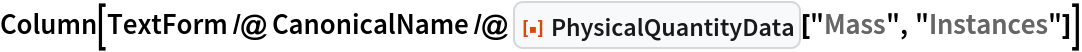Out=Display instances of length:

 In:=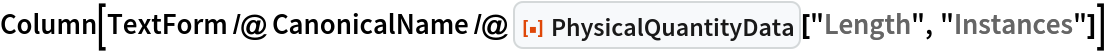Out=Get a random sample of 100 physical quantities out of all physical quantities:

 In:=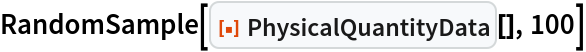Out=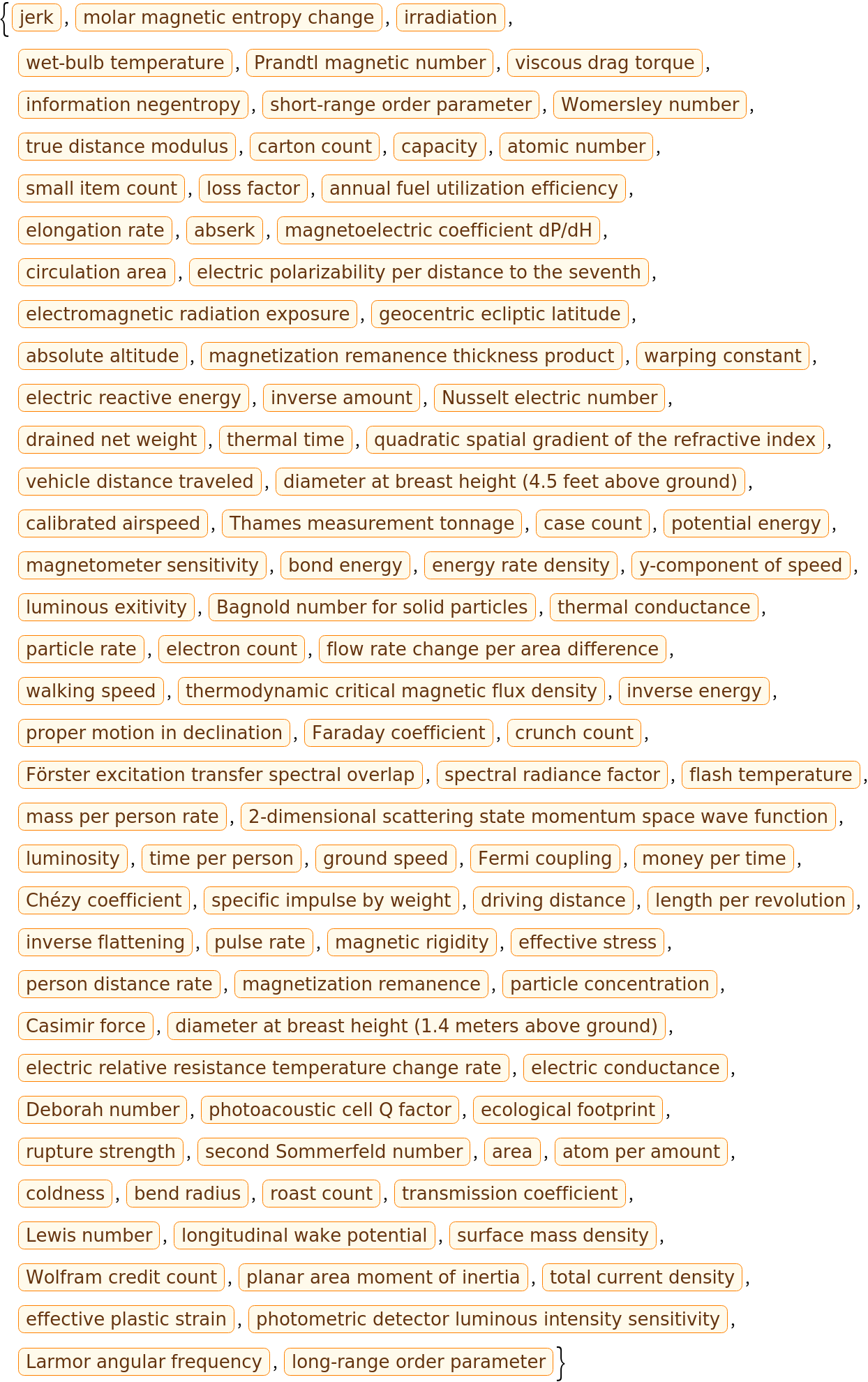Search for all names with "induct":

 In:=Out=Find the units of each quantity with the name induct:

 In:=Out=Peter Burbery

## Version History

• 1.0.0 – 06 September 2022